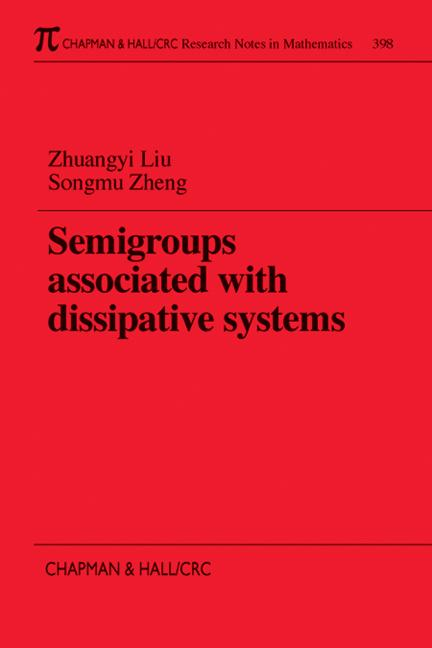# Semigroups Associated with Dissipative Systems

## 1st Edition

Chapman and Hall/CRC

224 pages

Paperback: 9780849306150
pub: 1999-01-29
SAVE ~\$37.00
Currently out of stock
\$185.00
\$148.00
x

FREE Standard Shipping!

### Description

Motivated by applications to control theory and to the theory of partial differential equations (PDE's), the authors examine the exponential stability and analyticity of C0-semigroups associated with various dissipative systems. They present a unique, systematic approach in which they prove exponential stability by combining a theory from semigroup theory with partial differential equation techniques, and use an analogous theorem with PDE techniques to prove analyticity. The result is a powerful but simple tool useful in determining whether these properties will preserve for a given dissipative system.

The authors show that the exponential stability is preserved for all the mechanical systems considered in this book-linear, one-dimensional thermoelastic, viscoelastic and thermoviscoelastic systems, plus systems with shear or friction damping. However, readers also learn that this property does not hold true for linear three-dimensional systems without making assumptions on the domain and initial data, and that analyticity is a more sensitive property, not preserved even for some of the systems addressed in this study.

### Reviews

"The book is clearly written and recommended especially for researchers and graduate students in the field of functional analysis, partial differential equations and control theory."

-Reinhard Racke, Mathematical Reviews

Preliminaries

Some Definitions

C0-Semigroup Generated by Dissipative Operator

Exponential Stability and Analyticity

The Sobolev Spaces and Elliptic Boundary Value Problems

Linear Thermoelastic Systems

The Setting of Problems for the One-Dimensional Thermoelastic System

The Exponential Stability for the Dirichlet Boundary Conditions at Both Ends

The Exponential Stability for the Stress-Free Boundary Conditions at Both Ends

The Exponential Stability for the Stress-Free Boundary Conditions at One End

The Thermoelastic Kirchhoff Plate Equations

Linear Viscoelastic System

Linear Viscoelastic System

Wave Equation with Locally Distributed Damping

Linear Viscoelastic System with Memory

The Linear Viscoelastic Kirchoff Plate with Memory

Linear Thermoviscoelastic Systems

Linear One-Dimensional Thermoviscoelastic System

Linear Three-Dimensional Thermoviscoelastic System with Memory

Elastic Systems with Shear Damping

Shear Diffusion Equations

Laminated Beam with Shear Damping

Linear Elastic Systems with Boundary Damping

Second-Order Hyperbolic Equation

Euler-Bernoulli Beam Equation

Uniformly Stable Approximations

Main Theorem

Approximations of the Thermoelastic System

Approximation of the Viscoelastic System

Bibliography